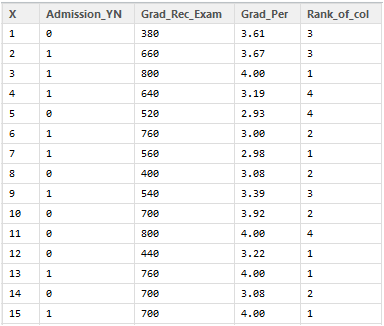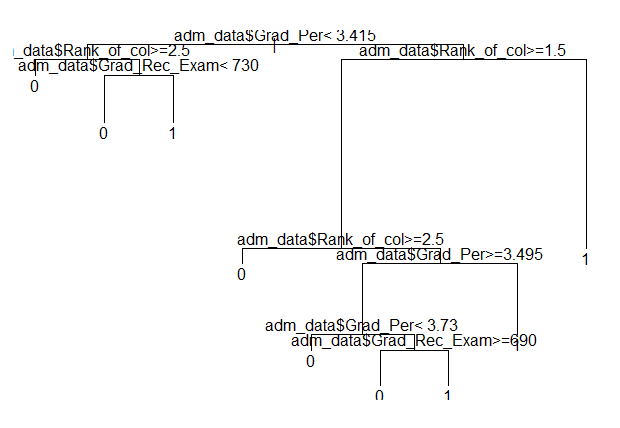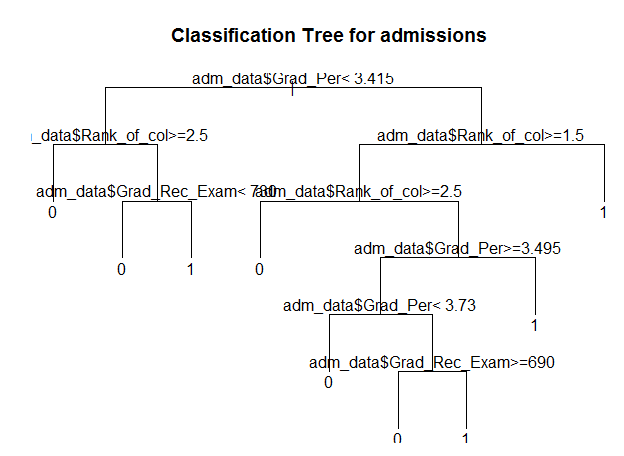# AnalyticBridge

A Data Science Central Community

Subscribe to DSC Newsletter

This blog talks about:

1. How to create a decision tree for the admission data.

2. Use rattle to plot the tree.

3. Validation of decision tree using the ‘Complexity Parameter’ and cross validated error.

4. Prune the tree on the basis of these parameters to create an optimal decision tree.

To understand what are decision trees and what is the statistical mechanism behind them, you can read this post : How To Create A Perfect Decision Tree

To create a decision tree in R, we need to make use of the functions rpart(), or tree(), party(), etc.

rpart() package is used to create the tree. It allows us to grow the whole tree using all the attributes present in the data.

`> library("rpart") > setwd("D://Data") > data <- read.csv("Gre_Coll_Adm.csv") > str(data)  'data.frame': 400 obs. of 5 variables:  \$ X : int 1 2 3 4 5 6 7 8 9 10 ...  \$ Admission_YN : int 0 1 1 1 0 1 1 0 1 0 ...  \$ Grad_Rec_Exam: int 380 660 800 640 520 760 560 400 540 700 ...  \$ Grad_Per : num 3.61 3.67 4 3.19 2.93 3 2.98 3.08 3.39 3.92 ...  \$ Rank_of_col : int 3 3 1 4 4 2 1 2 3 2 ... > View(data)``> adm_data<-as.data.frame(data) > tree <- rpart(Admission_YN ~ adm_data\$Grad_Rec_Exam + adm_data\$Grad_Per+ adm_data\$Rank_of_col,  + data=adm_data,  + method="class")`

rpart syntax takes ‘dependent attribute’ and the rest of the attributes are independent in the analysis.

Admission_YN : Dependent Attribute. As admission depends on the factors score, rank of college, etc.

Grad_Rec_Exam, Grad_Per, and Rank_of_col : Independent Attributes

rpart() returns a Decison tree created for the data.

If you plot this tree, you can see that it is not visible, due to the limitations of the plot window in the R console.

`> plot(tree) > text(tree, pretty=0)`Read the rest of it on Edureka.co

Views: 1310

Comment